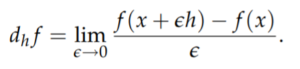# Gateaux Differential

The Gateaux differential is a generalization of the directional derivative. It is named after French mathematician René Gateaux, who was killed in WWI at age 25.

“Don’t worry about the name and notation. The Gateaux variation is just a directional derivative. It would be perfectly reasonable to write DhJ(x) instead of ∂J(x, h)” ~ Steve Cohn .

## Gateaux Differential Definition

The Gateaux Differential is a weak derivative defined on Banach spaces. Let f: V → U be a function, h ≠ 0 and x be vectors in V. Then :A function is Gateaux differentiable at point x only if all directional derivatives exist and form a bounded linear operator Df(x) : v ↦ ∂vf(x) .

## How Do I Calculate a Gateaux Differential?

At first it might seem complicated to calculate a Gateaux differential because each point h has more than one: In two dimensions there is one “forward” derivative and one “backward” one and in three or more dimensions there are an infinite number of differentials for each point h. However, when you calculate a Gateaux derivative you’re only calculating in one direction of h. The idea is similar to finding partial derivatives (where you can choose to find ∂/∂x or ∂/∂y). Therefore, although each point has multiple differentials, you can still calculate ordinary derivatives (using the chain rule and other rules for one-dimensional calculus) along a specified direction.

You do have to choose the direction of h with care. You can only take admissible variations—vectors hX where x + εh lies in the domain D for small ε .

## Fréchet vs. Gateaux

The Gateaux is directional (i.e., it coincides with derivatives in the direction of a vector), while the Fréchet derivative coincides with the full differential of f. Therefore, if the Fréchet exists, then the Gateaux exists. However, existence of a Fréchet derivative does not imply existence of a Gateaux derivative .

## References

 Cohn, S. The Gateaux Variation of a Functional. Retrieved November 23, 2021 from: https://www.math.unl.edu/~scohn1/8423/calcvar1.pdf
 Long, K. (2009). Math 5311 – Gateaux differentials and Frechet derivatives.
Retrieved November 23, 2021 from: https://www.math.ttu.edu/~klong/5311-spr09/diff.pdf
 Appendix A Gateaux and Frechet Differentiability. Retrieved November 23, 2021 from: https://link.springer.com/content/pdf/bbm%3A978-3-642-16286-2%2F1.pdf
 Slastikov, V. & Kitavtsev, G. (2017). Calculus of Variations.

CITE THIS AS:
Stephanie Glen. "Gateaux Differential" From StatisticsHowTo.com: Elementary Statistics for the rest of us! https://www.statisticshowto.com/gateaux-differential/